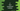# C program to find compound interest using user input values# C program to find compound interest using user given values :

In this C programming tutorial, we will learn how to find the compound interest for a time interval using user input values. The user will enter the values and our program will calculate and print out the compound interest.

The formulae to calculate compound interest is as below :

A = P (1 + R/(100 * n))nt

Here, A = Total amount of money i.e. Principal amount + compound Interest P = Principal amount or starting amount R = Rate of interest annually n = Number of compounding periods per year t = Total number of years

So, if we want to calculate the actual compound interest, we will have to deduct the principal amount from the final result :

``Compound Interest = A - P``

Let’s try to implement this using a C program :

``````#include <stdio.h>
#include <math.h>

int main()
{
//1
float P, R, n, t;
float finalAmount,compoundInterest;

//2
printf("Enter principal amount : ");
scanf("%f",&P);

printf("Enter rate of interest(Annually) : ");
scanf("%f",&R);

printf("Enter number of compounding periods per year : ");
scanf("%f",&n);

printf("Enter time in years : ");
scanf("%f",&t);

//3
finalAmount = P * pow((1 + R /(100 * n)),(nt));
compoundInterest = finalAmount - P;

//4
printf("Final amount : %f\n",finalAmount);
printf("Compound Interest : %f\n",compoundInterest);
return 0;
}``````

### Explanation :

The commented numbers in the above program denote the step numbers below :

1. Create different float variables to store the principal amount, rate of interest annually, the number of compounding periods, time in year, final amount and compound interest generated.
2. Ask the user to enter all values. Read and store it in the variables.
3. Find out the final amount and compound interest using the same formulae we have explained before.
4. Finally, print out the final amount and compound interest to the user.

Now, let’s check a few running examples of the above program :

``````Enter principal amount : 1000
Enter rate of interest(Annually) : 20
Enter number of compounding periods per year : 1
Enter time in years : 4
Final amount : 2073.600342
Compound Interest : 1073.600342

Enter principal amount : 1000
Enter rate of interest(Annually) : 20
Enter number of compounding periods per year : 2
Enter time in years : 4
Final amount : 2143.589111
Compound Interest : 1143.589111

Enter principal amount : 2000
Enter rate of interest(Annually) : 5
Enter number of compounding periods per year : 1
Enter time in years : 10
Final amount : 3257.787842
Compound Interest : 1257.787842``````

# Conclusion :

In the examples above, you can see that the first and the second one is almost the same, only the number of compounding periods per year is different. Even if you have the same capital and yearly interest rate, if the compounding periods are different, interest will be different for the same period of time.

Try to run the above examples and drop one comment below if you have any queries.

You might also like :